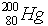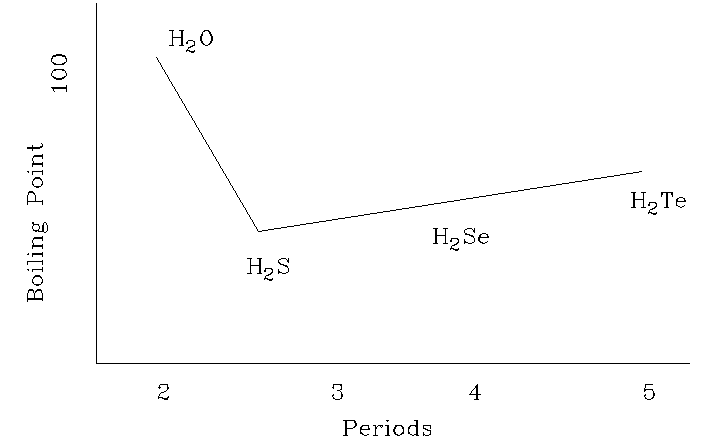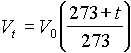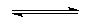SCHOLATIC APTITUDE TEST 1996

CHEMISTRY

 Time: 1 Hour Max.Marks : 60

Instructions:

(1) Answer must be written either in English or the medium of instruction of the candidate in high school.

(2) There will be no negative marking

(3) There are THREE Sections. Answer all questions of a section at one place

(4) Use of calculators or graph papers is not permitted

SECTION-I

Fill in the Blanks (20 x 1 = 20 Marks)

1. The actual species exist in the aqueous solution of H2SO4 are ____________________________

2. He, Ne, Ar, Kr, Xe are called inert gases, but to create inert conditions during the chemical reactions nitrogen gas is used. The inert behaviour of nitrogen is due to ____________________

3. pH of the aqueous solution increases with dilution. pH of the solution increases by one unit on 10 fold dilution. One litre of solution of pH=6 is diluted to 100 litres pH of the resulted solution is nearly _______________

4. Four quantum numbers of the electron is useful in determining the spatial position of the electron in given atom. The four quantum numbers of the differentiating electron in scandium atom (Sc=21) is ______________

5. Radio activity is the property of instable nucleus. Atoms with instable nucleus emits a , b , g radiations. The instability of the nucleus is due to __________________

6. Bond dissociation energy of the Cl2 is greater than that of F2 as well as Br2.This is due to ________

7. Zinc decolourises the blue colour of aqueous blue vitriol, but silver does not do so. This is because ___________________

8. Every atom and ion contain three fundamental particles. The difference between the mercury () atom and mercurous ion is ______________ (in terms of number of fundamental particles)

9. Chemical formula of the compound which is commonly used to detect presence of Cl- ion in drinking water is _____________________ and this compound can be detected by using ______________ aqueous solution

10. Most of the elements exist in the nature only in the form of compounds this is due to ___________

11. __________________________ is used to detect the presence of water.

12. When a compressed gas at a temperature is allowed to expand through a small porous plug or small orifice the temperature of gas ________________________

13. Self addition to form macro molecules is characteristic reaction exhibited by many unsaturated compounds. PVC obtained by ______________________

14. During chemical bonding BF3 molecule always accepts pair of electrons to form a bond. This is due to __________________

15. Allotropy is the phenomenon of an element in which the element shows different physical properties and similar chemical properties. This is due to _______________________________

16. Be, Mg, Ca, Sr and Ba metals are called alkaline earth metals. This is due to _________________

17. A covalent bond is formed by the equal sharing of electrons from the bonded atoms. The actual forces responsible for holding the atoms together in the molecule are _______________________

18. According to collision theory rate of a reaction is directly proportional to the number of effective collisions per second. The meaning of the effective collisions is __________________________

19. _________________________ is used as a flux in blast furnace to remove _________________ in the extraction of iron

20. Theoretically the orbitals present in hydrogen atom are __________________________

SECTION-II

 Explain the following: (10 x 21/2 = 25 Marks)

1. Some chemical reactions are reversible and some are irreversible.

2. The boiling points of hydrogen compounds of oxygen family can be represented graphically as follows:3. Solubility is generally defined as the number of grams of the solute present in 100 gm of the solvent at a given temperature. Generally as the temperature increases solubility increases but in few cases it decreases with increase of temperature.

4. Molality of the solution is independent of temperature where as molarity is dependent on temperature.

5. According to the equation, when t = -2730C Vt becomes zero. i.e. a gas would occupy no volume at all

6. What type of graphs are obtained for P vs V under isothermal conditions of T1, T2, and T3 where T3 > T2 > T1. To compare draw them in a single figure.

7. Only under certain conditions real gases obey the Boyle’s law.

8. Generally rate of the chemical reaction exponentially decreases with time.

9. pH of the 0.1 M HCl is 1, but 0.1 M acetic acid solution is greater than 1.

10. Glass is called as supercooled liquid.

SECTION-III

 Solve the Problems: (15 Marks)

1. 6.3 gm of oxalic acid crystals (C2O4H2.2H2O) in 250 ml flask is treated with excess of concentrated sulphuric acid. Heat the flask gently. The two gas components which are released passed through the caustic soda. Finally one gas is collected over water.

a) what is the function of concentrated H2SO4?

b) what are the gas components ?

c) what gas component is absorbed by caustic soda?

d) if the absorption is chemical process, write the chemical equation

e) how many grams of each component is released?

f) what is the pressure of the gas collected over water, if the volume of the gas is 2 litres and temperature of gas is 270C (aqueous tension = 30 mm Hg at 270C). ( 6 )

2. When salt `A’ is treated with concentrated H2SO4 , `B’ (solid) and `C’ (gas) are formed. `C’ forms white fumes with NH3 . `B’ is treated with coke and lime stone and heated to 8000C, a solid `D’ is formed. `D’ is treated with water to obtain a solution E and a solid F. When excess CO2 gas is passed through this solution precipitate `G’ is formed. `G’ on strong heating gives `E’. Write the sequence of chemical reactions and identify the substances from A to G. ( 3 )

3. 0.1525 gm of an unknown liquid on evaporation displaces 35.05 ml of air and collected in a graduated glass burette. The pressure of displaced air is 730 mm Hg and the temperature is 200C. Calculate the molecular weight of the liquid. ( 3 )

4. In the chemical reaction 2X+3Y4Z

1.0 mole of X, 1.25 mole of Y and 2.5 mole of Z are found at equilibrium in a 1.0 litre reaction mixture.

a) calculate the value for K

b) calculate the value for K if the same mixtrue had been found in a 2.0 litre vessel.      ( 3 )